V2EX = way to explore
V2EX 是一个关于分享和探索的地方

V2EX  ›  分享创造

# 写给前端工程师看的函数式编程对话（系列文章）

FrankFang128 · 2021-03-14 12:52:44 +08:00 · 4080 次点击

# 第 0 天

## 第一天：重新开始学写代码

``````for(let i = 0; i< array.length; i++){
console.log(array[i])
}
``````

``````for(let key in array){
console.log(array[key])
}
``````

``````array.forEach(item => console.log(item))
``````

``````forEach(array, item => console.log(item))
``````

``````let array = ['a','b','c']
let forEach = (array, fn) => {
if(array.length === 0) return
fn(array)
forEach(array.slice(1), fn)
}
forEach(array, item => console.log(item))
``````

1. 程序员应该记住性能好的代码，就算放弃可读性也值
2. 程序员以可读性为优先，让编译器想办法优化性能

reverse = (string) => {
if(string.length <= 1){return string}
let last = string[string.length-1]
let head = string.substr(0, string.length - 1)
return last + reverse(head) // 把最后一个字符移到前面，然后递归
}

/*1*/ quickSort = (array) => {
/*2*/ if(array.length <= 1) {return array}
/*3*/ let [pivot, ...rest] = array
/*4*/ let small = rest.filter(i => i<=pivot)
/*5*/ let big = rest.filter(i => i>pivot)
/*6*/ return [...quickSort(small), pivot, ...quickSort(big) ]
/*7*/ }

## 第二天：递归 & 尾递归

``````f = n => {
if(n<=1) {
return 1
} else {
return n * f(n-1)
}
}
``````

``````f(4)
= 4 * f(3)
= 4 * (3 * f(2))
= 4 * (3 * (2 * f(1)))
= 4 * (3 * (2 * 1))
= 4 * (3 * 2)
= 4 * 6
= 24
``````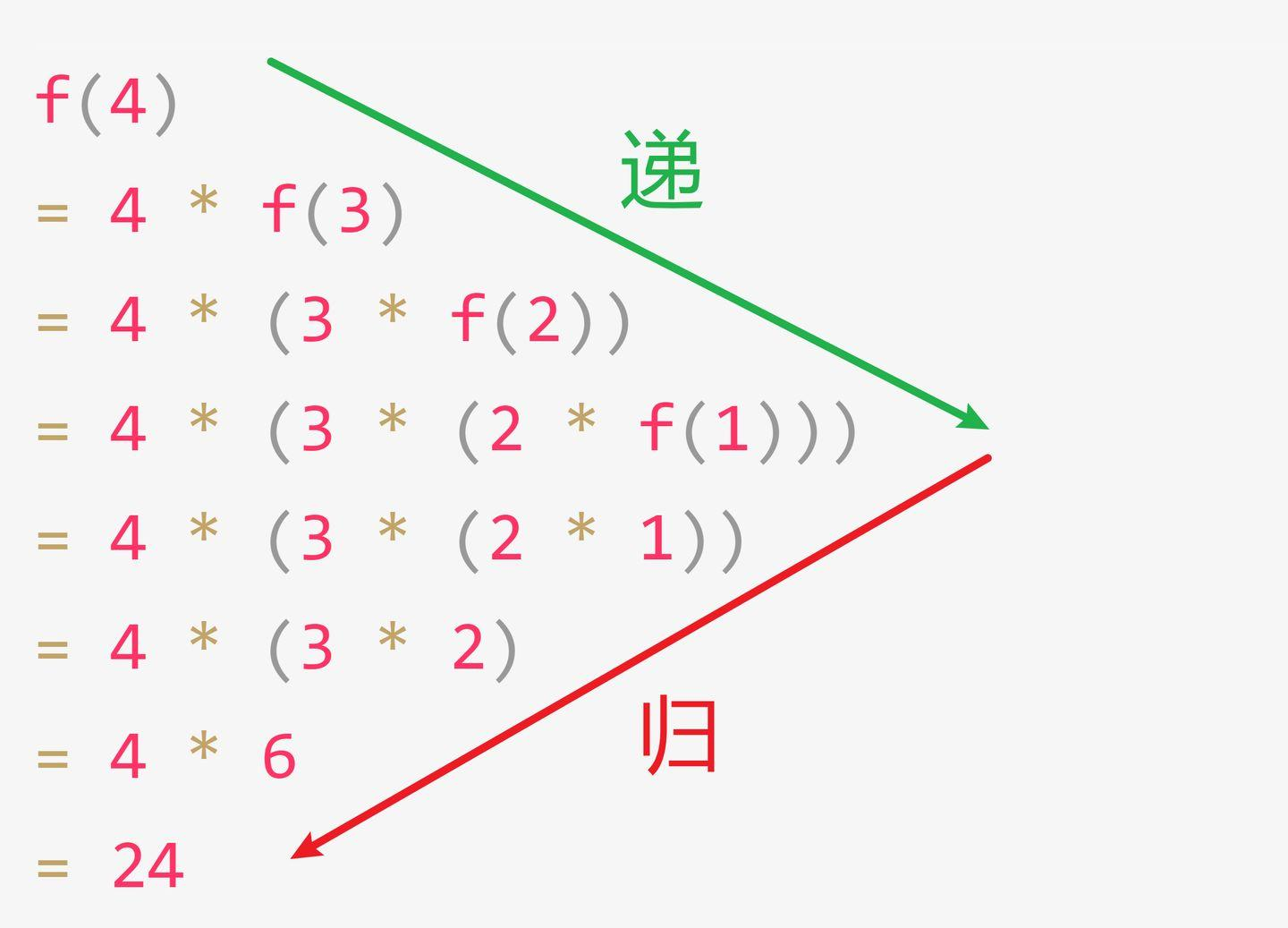``````print = (n) => {
if(n===0){return}
console.log(n)
return print(n-1) // 此处 return 可以省略不写，因为没有返回值
}
``````

``````print(4)
= print(3)
= print(2)
= print(1)
= 结束
``````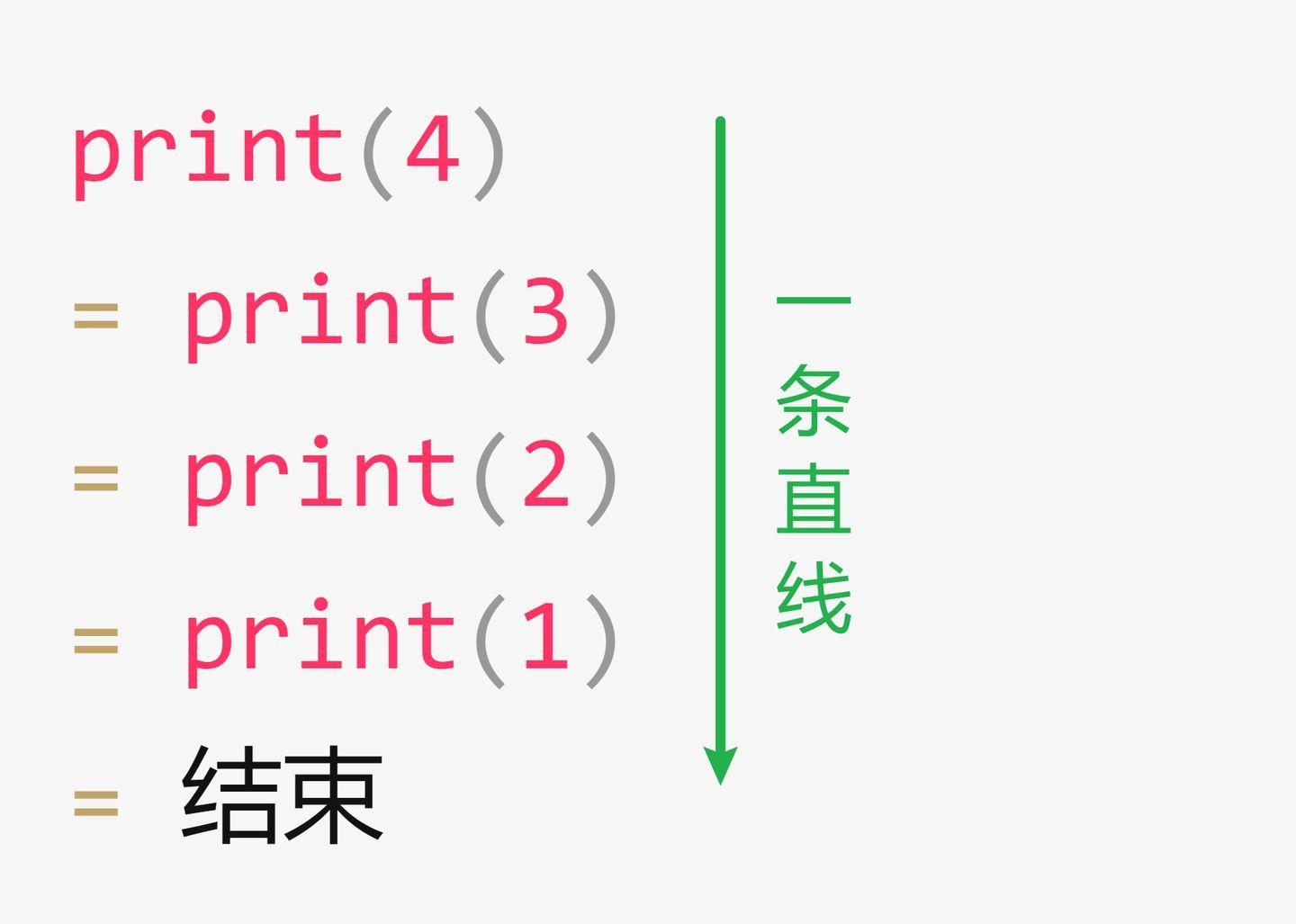``````f = (n, result = 1) =>
n > 1 ? f(n-1, result * n) : result
``````

``````f(4)
= f(3, 4)
= f(2,12)
= f(1,24)
= 24
``````

``````fib = (n) =>
n === 0 ? 0 :
n === 1 ? 1 :
fib(n-1) + fib(n-2)
``````

``````fib(4)
= f(3) + f(2) // 简写为 f
= (f(2) + f(1)) + (f(1) + f(0))
= ((f(1) + f(0)) + f(1)) + (f(1) + f(0))
= ((f(1) + f(0)) + f(1)) + (1 + 0)
= ((f(1) + f(0)) + f(1)) + 1
= ((f(1) + f(0)) + 1) + 1
= ((1 + 0) + 1) + 1
= (1 + 1) + 1
= 2 + 1
= 3
``````

``````fib = (n, prevResult = 0, result = 1) =>
n === 0 ? prevResult :
n === 1 ? result :
fib(n - 1, result, prevResult + result)
``````

``````fib(4)
= fib(3, 1, 1)
= fib(2, 1, 2)
= fib(1, 2, 3)
= 3
``````

``````/*1*/ quickSort = (array) => {
/*2*/   if(array.length <= 1) {return array}
/*3*/   let [pivot, ...rest] = array
/*4*/   let small = rest.filter(i => i<=pivot)
/*5*/   let big = rest.filter(i => i>pivot)
/*6*/   return [...quickSort(small), pivot, ...quickSort(big) ]
/*7*/ }
``````

1. quickSort(small) 之后必须要回头执行 quickSort(big)
2. quickSort(big) 执行完了之后必须要回头把 quickSort(small)、pivot、quickSort(big)拼接起来

``````/*1*/ qs = (array, next) => {
/*2*/     if(array.length <= 1) { return next(array)}
/*3*/     const [pivot, ...rest] = array
/*4*/     const small = rest.filter(i=>i<=pivot)
/*5*/     const big = rest.filter(i=>i>pivot)
/*6*/     qs(small, (sortedSmall)=>{
/*7*/         qs(big, (sortedBig)=>{
/*8*/             next([...sortedSmall, pivot, ...sortedBig])
})
})
}

qs([1,10,5,2,7,3,8,4,9,6], (result)=>{
console.log(result)
})

// 输出 [1, 2, 3, 4, 5, 6, 7, 8, 9, 10]
``````

``````/*4*/ let small = rest.filter(i => i<=pivot)
/*5*/ let big = rest.filter(i => i>pivot)
/*6*/ return [...quickSort(small), pivot, ...quickSort(big) ]
``````

``````/*6*/ qs(small, (sortedSmall)=>{
/*7*/   qs(big, (sortedBig)=>{
/*8*/     next([...sortedSmall, pivot, ...sortedBig])
``````

1. 递归（recursion）有一种特殊的形式，叫做「尾递归」
2. 递归版阶乘可以写成「尾递归」，方法是把上一次的结果传给下一次调用
3. 递归版斐波那契可以写成「尾递归」，方法是把上两次的结果传给下一次调用
4. 递归版快排可以写成「尾递归」，方法是把后续操作写成一个函数，传给下一次调用（即 CPS）
5. 补充一点：递归改写成尾递归还有其他方法，并不只是我上课讲的这么一点

# 第三天：从 JS 函数到 Haskell 函数

``````add = (a, b) => a + b
``````

``````c_add = (a) => (b) => a + b
``````

``````temp = c_add(1)
temp(2) // 得到 3
``````

``````c_add(1)(2) // 得到 3
``````

``````add = (a, b) => a + b
c_add = (a) => (b) => a+b
``````

``````add(1,2) // 3

``````

``````multi = (a, b, c) => a * b * c
c_multi = a => b => c => a * b * c
``````

``````anyFn = (p1, p2, ..., pn) => doSomethingWith(p1, p2, ..., pn)
c_anyFn = p1 => p2 => ... => pn => doSomethingWith(p1, p2, ..., pn)
``````

``````template = Handlebars.compile("<div>{{user.name}}</div>"); // 传第一个参数

html1 = template({user:{name:'frank'}}) // 传第二个参数
// html1 为 <div>frank</div>
html2 = template({user:{name:'jack'}}) // 传第二个参数
// html2 为 <div>jack</div>
``````

``````type Add = (a: number, b: number) => number;
// 改成单参
type Add = (a: number) => (b: number) => number;
``````

``````const add = a => b => fn => fn(a+b)
add(1)(2)( (result)=> result*2 ) // 得到 6
``````

``````type Add = ...
``````

``````type Add = (a:number) => (b:number) => ...
``````

``````type Add = (a:number) => (b:number) => (fn: (c:number) => number ) =>
``````

``````type Add = (a:number) => (b:number) => (fn: (c:number) => number) => number
``````

``````doubleMe :: Int -> Int
doubleMe x = x * 2
``````

``````  putStrLn (show (doubleMe 100))
``````

``````putStrLn  (show  (doubleMe 100))

putStrLn \$ show \$ doubleMe 100
``````

``````let n = doubleMe 100
let string = show n
putStrLn string
``````

``````doubleMe :: Int -> Int
doubleMe x = x * 2

main :: IO ()
main = putStrLn \$ show \$ doubleMe 100
``````

34 条回复    2022-01-29 13:23:43 +08:00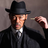1 FrankFang128   OP   2021-03-14 13:30:20 +08:00 方：我跟你举个例子，你应该知道 ++i 比 i++ 的性能要好很多吧？学生：哦？为什么？方：原因不重要，你可以看看[这篇文章]( https://www.omegaxyz.com/2017/05/20/aboutippandppi/)，也可以不看。假设你已经知道 ++i 比 i++ 性能要好很多，请问，你在写 for 循环时，写 i++ 还是写 ++i 。学生：我以前一直写 i++，听你这么一说，我是不是应该写 ++i方：不用，因为编译器帮你优化了，编译器一旦发现 for 循环的第三个语句是 i++，会自动优化为 ++i，所以不需要程序员自己记住这么多规则，网上这种乱七八糟的优化技巧太多了，你能记住几个学生：编译器这么智能！方：你也不想想，写编译器的那帮人比写 JS 的人智商高多少。学生：也是方：甚至有的时候，你为了提高性能而采用的怪异写法，会降低程序的性能，因为它得不到编译器的优化。编译器一般会优化常见的写法。学生：原来是这样方：所以程序员应该尽量写符合社区习惯的代码，只在性能瓶颈处手动优化性能。但是由于 JS 和 Java 这帮人常年的「教育」，像你这样的新人已经都认为应该尽量不用「递归」，所以 JS 和 Java 的编译器没有必要花时间优化小众写法，优化之后反而会造成安全和 debug 相关的问题。学生：那说递归「开销大」和「浪费内存」是不是也是类似的误区。方：是的，如果某个语言特性被程序员用得多，编译器就会想尽办法让它变快。现在，你可以放弃你对递归的偏见了吗？学生：可以，那我是不是还得放弃 JS 和 Java 的编译器？方：是的，这两门语言的社区文化与函数式不太兼容，而且主流版本的编译器是不支持这些优化的，也许未来的版本有。学生：那我再多嘴问一句，「递归」没有缺点吗？方：也有，以后会讲，但是瑕不掩瑜，利大于弊。学生：好，我先记下来，虽然还没有完全被说服。方：我们是从哪聊到这的？哦，是从「你没法第一时间给出递归的写法」聊到这的。那么我给你出第二题，写一个将字符串反转的函数，不能违反「数据不可变」的约定哦。学生：递归写法我会 reverse = (string) => { if(string.length <= 1){return string} let last = string[string.length-1] let head = string.substr(0, string.length - 1) return last + reverse(head) // 把最后一个字符移到前面，然后递归 } 方：写得还挺快学生：丢下偏见之后果然写得快很多，不过还是觉得效率会慢、内存会浪费方：过几天你就习惯了，我也会教你怎么优化。再来一个，快速排序学生：简单 /*1*/ quickSort = (array) => { /*2*/ if(array.length <= 1) {return array} /*3*/ let [pivot, ...rest] = array /*4*/ let small = rest.filter(i => i<=pivot) /*5*/ let big = rest.filter(i => i>pivot) /*6*/ return [...quickSort(small), pivot, ...quickSort(big) ] /*7*/ } 学生：这个快排写得是真的爽，但我还是担心浪费内存，第 3 、4 、5 、6 行全是内存复制方：哼，那是因为 JS 和 Java 的数据可变，所以不能直接复用数据啊。如果数据是不可变的，`let [pivot, ...rest] = array` 可以直接复用内存啊，rest 和 array 复用同一片内存问题不大学生：好像是这么回事，那 rest.filter(i => i<=pivot) 呢方：这个内存很难优化。但是你要知道，你用函数式写只用 5 行代码，用指令式写可能要 20 行代码，函数式追求的是逻辑上的简洁，先让逻辑变美，然后等遇到性能瓶颈了再上优化学生：好吧方：等下，你怎么好像对「数据不可变」还是没有完全接受啊，总想着改原来的内存？学生：才第一天嘛，过几天我才能适应方：好吧，今天就先到这里，明天继续。2 FrankFang128   OP   2021-03-14 13:33:01 +08:00 大家请忽略 1 楼，1 楼内容是我发错了。3 FrankFang128   OP   2021-03-14 18:58:15 +08:00 @Livid 能把一楼删掉吗？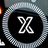4 cmdOptionKana      2021-03-14 20:05:50 +08:00 干货啊5 GeruzoniAnsasu      2021-03-14 20:38:13 +08:00 我以为 “写给前端” 会从 “你怎么让元素 B 的颜色随着按钮 A 的位置移动而改变” 这种角度入门之前有一个帖子问 怎么实现对象值之间的相互约束，比如定义 C=A+B，改变 AB 的值能直接导致 C 结果改变。这种具体场景才好解释函数式的特点和必要性写给前端为啥不直接讲讲怎样 “make something reactive” ?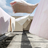6 mmtromsb456      2021-03-14 20:44:31 +08:00 希望楼主做个汇总版放 blog 之类的地方,支持关注一下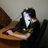7 AEDaydreamer      2021-03-14 22:16:29 +08:00 我最近正好在看 functional programming in JavaScript 相关的内容 ,受益良多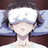8 lbyo      2021-03-15 09:54:27 +08:00 ++i 和 i++ 是有区别的吧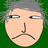9 yunyuyuan      2021-03-15 10:18:09 +08:00 好10 jinliming2      2021-03-16 01:22:11 +08:00 via iPhone JS ES6 提案有尾调优化，但目前好像只有用 Webkit 的 Safari 支持了，其他的 Chrome 和 Firefox 都还没实现。11 FrankFang128   OP   2021-03-16 03:22:18 +08:00 @jinliming2 后来取消了这个提案12 namelosw      2021-03-16 09:33:43 +08:001 @jinliming2 一开始是 Safari 和 Chrome 实现了, 然后开会的时候 Mozilla 说我们还没做, Edge 做不了不做了.最后就进行不下去了…然后 Chrome 一看算了, 反正别人也不做, 就把自己的 TCO 也删了, 现在好像只有 Safari 的还留着.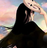13 abersheeran      2021-03-17 09:51:10 +08:00 你这个“编译器应当优化尾递归”的说法放在 Py 里可能可以，但是放在 JS 里绝不可能的。JS 的历史包袱可是最重的。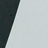14 huabalance      2021-03-17 10:59:33 +08:00 醍醐灌顶，期待更新。15 FrankFang128   OP   2021-03-18 11:11:18 +08:00 @huabalance 已更新16 FrankFang128   OP   2021-03-18 12:26:09 +08:00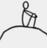17 sillydaddy      2021-03-19 17:04:49 +08:00 “递”“归”解释的很形象哦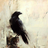18 cyrbuzz      2021-03-20 22:23:09 +08:00 递归最大的缺点是不是性能不高？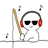19 Wincer      2021-03-20 22:45:23 +08:00 via Android 不错，有种让我觉得在读《冒号课堂》的感觉。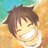20 Chingim      2021-03-20 22:53:05 +08:00 via iPhone1 写得挺好的，关注了（一般我不夸人21 FrankFang128   OP   2021-03-20 23:12:35 +08:001 @cyrbuzz 唉，你白看了 :(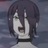22 NPC666      2021-03-21 20:31:34 +08:00 厉害厉害，醍醐灌顶23 xml123      2021-03-22 18:14:13 +08:001 难得一见的教程贴，感谢 lz，只可惜 v2 这个平台不太适合做这种连载性质的内容24 Chingim      2021-03-23 20:28:07 +08:00 用函数式的思想编写 js 代码, 有两个问题很困惑, 请先生教我:1. 提倡纯函数, 导致函数的参数特别长. 更恼人的是, 层层调用的函数, 虽然只有最里层的函数需要某个参数, 外面 n 层的函数的参数列表都不得不加上这个参数. 这种场景如何解决2. 如何隔离副作用. 一个典型的前端场景: 获取远端数据, 渲染到 dom 节点上, 点击刷新按钮, 重新获取数据进行渲染, 几乎都是副作用. 这个场景下的函数式代码是长什么样子的?25 FrankFang128   OP   2021-03-24 01:11:38 +08:00 @Chingim 1. Haskell 会自动简化这种情况，f a b c d 等价与 f(a)(b)(c)(d) 所以你需要换一门语言 2. React 的 useEffect 就是隔离副作用的例子，它让副作用全部都在 useEffect 的第一个参数里26 FrankFang128   OP   2021-03-24 01:16:17 +08:00 @Chingim 你说的 「虽然只有最里层的函数需要某个参数, 外面 n 层的函数的参数列表都不得不加上这个参数」这个问题指令式编程语言也存在，比如 C 语言的 f(null, null ,null, null, null, 'hi') 为了传 'hi' 不得不先传 5 个 null，指令式编程的解决办法是把所有参数都绑到对象上，比如 obj.a = 'a'; obj.b = 'b'; obj.addAB()；那么函数式也有对应的写法 const afterCallA = f(a); const afterCallB = afterCallA(b); const getAB = afterCallB()我不清楚我回答的是不是你问的，如果有代码例子给我看就更好了。27 Chingim      2021-03-24 11:16:14 +08:0028 FrankFang128   OP   2021-03-24 13:44:52 +08:0029 Chingim      2021-03-24 15:03:07 +08:00 @FrankFang128 懂了, 就是把“传递 inner 函数需要的参数”变成“传递 inner 函数”谢谢30 DrakeXiang      2021-03-25 23:41:41 +08:00 我的思路真的是跟学生一模一样。。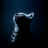31 DaguguJ      2021-03-26 12:16:28 +08:00 讲得 lisp 的编程风格，对吧32 cyrbuzz      332 天前 半年后又读了几遍，收益很多，感谢~。33 vaporSpace      243 天前 受益良多34 cjydawn      194 天前 受益良多，感谢